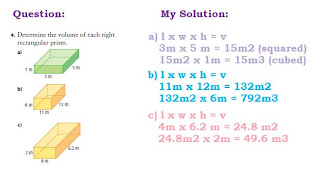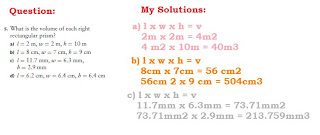## Thursday, February 17, 2011

### Melanie's Volume Scribe Post

For my post, I will be answering the questions 4, 5, and 6 on the textbook work we did in class today. ( pages 258 - 261 )*Sorry if some of the pictures are hard to see, just click it and it will appear bigger.

*Here is a link that will hopefully help those of you who don't understand how to find the volume of a rectangle / square.

If you guys don't

Cylinder Volume and Volume Problemsv= π x r x r
v= 3.14 x 12cm x 12 cm
v= 452.16 cm2 x 15
v= 6782.4 cm3

6782.4 cm3 / 4= 1695.6 cm3

The volume of cheese taken from the block was 1695.6 cm3.
One assumption I made was that they cut 1/4 from the cheese.r= d/2
r= 0.8m/2
r= 0.4 m

v=
π x r x r
v= 3.14 x 4cm x 4cm
v= 0.5024 cm2 x 10cm
v= 5.024 m3

r= d/2
r= 1m/2
r= 0.5m

v= π x r x r
v= 3.14 x 0.5m x 0.5m
v= 0.785 m2 x 10m
v= 7.85 m3

7.85 m3 - 5.024 m3 = 2.826 m3

The volume of concrete required to make the culvert is 2.826 m3.

Here is my volume video:

1.2.3.